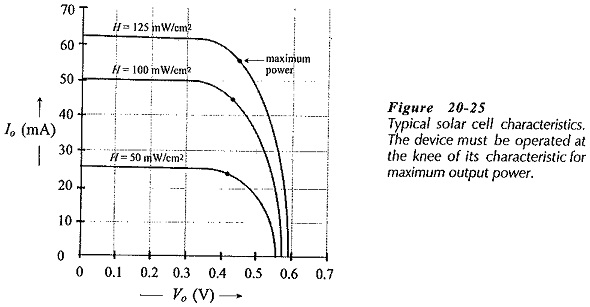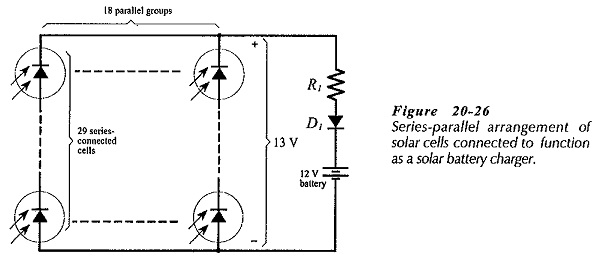## Solar Energy Conversion Diagram:

The Solar Energy Conversion Diagram, or solar energy converter, is essentially a large photodiode designed to operate solely as a photovoltaic device and to give as much output power as possible. To provide maximum output current, solar cell surface areas are much larger than those of signal photodiodes,

Typical solar cell output characteristics are illustrated in Fig. 20-25. Consider the characteristic for a 100 mW/cm2 illumination level. If the cell is short-circuited, the output current (Io) is 50 mA. Because the cell voltage (Vo) is zero at this point, the output power (Po) is zero.

Open-circuiting the cell gives Vo ≈ 0.55 V, but Io = O. So, Po is again zero. At the knee of the characteristic Vo ≈ 0.44 V and Io = 45 mA; giving Po = 20 mW. Therefore, for maximum output power, the device must be operated on the knee of the characteristic. As in the case of all other devices, the power must be derated at high temperatures.Figure 20-26 shows a group of series-parallel connected solar cells operating as a battery charger. Several cells must be series connected to produce the required output voltage, and several of these series-connected groups must be connected in parallel to provide the necessary output current.Scroll to Top